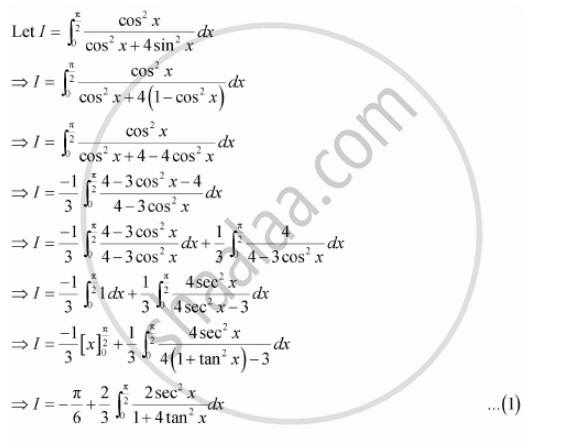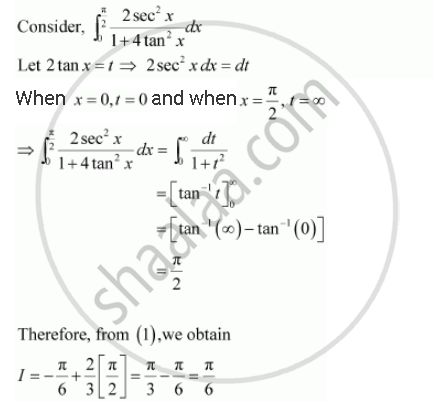Share
Notifications

View all notifications
Books Shortlist
Your shortlist is empty

# Solution for Evaluate the Definite Integrals Int_0^(Pi/2) (Cos^2 X Dx)/(Xos^2 X + 4 Sin^2 X) - CBSE (Science) Class 12 - Mathematics

Login
Create free account

Forgot password?
ConceptDefinite Integral as the Limit of a Sum

#### Question

Evaluate the definite integrals  int_0^(pi/2) (cos^2 x dx)/(xos^2 x + 4 sin^2 x)

#### SolutionIs there an error in this question or solution?

#### Video TutorialsVIEW ALL 

Solution Evaluate the Definite Integrals Int_0^(Pi/2) (Cos^2 X Dx)/(Xos^2 X + 4 Sin^2 X) Concept: Definite Integral as the Limit of a Sum.
S